# Diagonals

Draw a square ABCD whose diagonals have a length of 6 cm

a =  4.2426 cm

### Step-by-step explanation: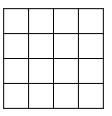Did you find an error or inaccuracy? Feel free to write us. Thank you!Tips to related online calculators

#### You need to know the following knowledge to solve this word math problem:

We encourage you to watch this tutorial video on this math problem:

## Related math problems and questions:

• Draw a trapezoidDraw a trapezoid if given a = 7 cm, b = 4 cm, c = 3.5 cm, diagonal AC = 5cm. Solve as a construction task.
• Trapezoidal prismCalculate the surface of the quadrilateral prism ABCDA'B'C'D 'with the trapezoidal base ABCD. The height of the prism is 12 cm; ABCD trapezoidal data: AB base length is 8 cm, CD base length is 3 cm, BC arm length is 4 cm, and AC diagonal length is 7 cm. L
• Construct rhombusConstruct rhombus ABCD if given diagonal length | AC | = 8cm, inscribed circle radius r = 1.5cm
• Complete constructionConstruct triangle ABC if hypotenuse c = 7 cm and angle ABC = 30 degrees. / Use Thales' theorem - circle /. Measure and write down the length of legs.
• Diagonal in rectangle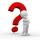In that rectangle ABCD is the center of BC point E and point F is center of CD. Prove that the lines AE and AF divide diagonal BD into three equal parts.
• Construct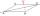Construct a rhombus ABCD, if the size of the diagonal AC is 6 cm and diagonal BD 8 cm long.
• Rhombus constructionConstruct ABCD rhombus if its diagonal AC=9 cm and side AB = 6 cm. Inscribe a circle in it touching all sides...
• Trapezium diagonalsIt is given trapezium ABCD with bases | AB | = 12 cm, |CD| = 8 cm. Point S is the intersection of the diagonals for which |AS| is 6 cm long. Calculate the length of the full diagonal AC.
• Draw a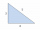Draw a triangle ABC, if you know: alpha = 60° side b = 4 cm side a = 10 cm
• Square gridSquare grid consists of a square with sides of length 1 cm. Draw in it at least three different patterns such that each had a content of 6 cm2 and circumference 12 cm and that their sides is in square grid.
• Triangle SSAConstruct a triangle ABC if |AB| = 5cm va = 3cm, CAB = 50 °. It is to create the analysis and construction steps.
• Diagonals at right angleIn the trapezoid ABCD, this is given: AB=12cm CD=4cm And diagonals crossed under a right angle. What is the area of this trapezoid ABCD?
• Draw triangle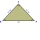Construct an isosceles triangle ABC, if AB = 7cm, the size of the angle ABC is 47°, arms | AC | = | BC |. Measure the size of the BC side in mm.
• Right triangleDraw a right triangle ABC if |AB| = 5 cm |BC| = 3 cm, |AC| = 4 cm. Draw Thales circle above the hypotenuse of the triangle ABC.
• Construct 1Construct a triangle ABC, a = 7 cm, b = 9 cm with right angle at C, construct the axis of all three sides. Measure the length of side c (and write).
• Construction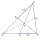Construction the triangle ABC, if you know: the size of the side AC is 6 cm, the size of the angle ACB is 60° and the distance of the center of gravity T from the vertex A is 4 cm. (Sketch, analysis, notation of construction, construction)
• Rhombus MATHConstruct a rhombus M A T H with diagonal MT=4cm, angle MAT=120°